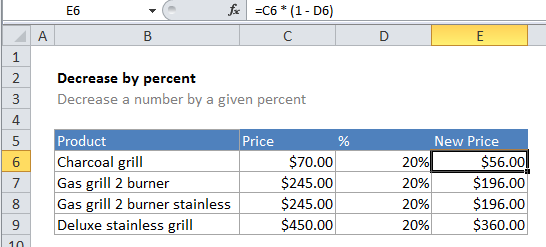## Excel Office

Excel How Tos, Tutorials, Tips & Tricks, Shortcuts

# How to calculate decrease by percentage in Excel

If you need to decrease a number by a certain percentage, you can use a simple formula that multiplies the number times the percent – 1.

## Formula

`=number*(1-percent)`